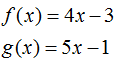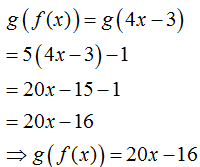# Given f(x)=4x -3 and g(x) = 5x-1 evaluate the composite function g[f(0)]

Question
1 views

Given f(x)=4x -3 and g(x) = 5x-1 evaluate the composite function g[f(0)]

check_circle

Step 1

Given functions areStep 2

The composition is given as...

### Want to see the full answer?

See Solution

#### Want to see this answer and more?

Solutions are written by subject experts who are available 24/7. Questions are typically answered within 1 hour.*

See Solution
*Response times may vary by subject and question.
Tagged in